xLenz's lawEncyclopedia
Lenz's law ˈ is a common way of understanding how electromagnetic circuits must always obey Newton's third law and The Law of Conservation of Energy
Conservation of energy
The nineteenth century law of conservation of energy is a law of physics. It states that the total amount of energy in an isolated system remains constant over time. The total energy is said to be conserved over time...

. Lenz's law is named after Heinrich Lenz
Heinrich Lenz
Heinrich Friedrich Emil Lenz was a Russian physicist of Baltic German ethnicity. He is most noted for formulating Lenz's law in electrodynamics in 1833....

, and it says:
An induced electromotive force
Electromotive force
In physics, electromotive force, emf , or electromotance refers to voltage generated by a battery or by the magnetic force according to Faraday's Law, which states that a time varying magnetic field will induce an electric current.It is important to note that the electromotive "force" is not a...

(emf) always gives rise to a current whose magnetic field opposes the original change in magnetic flux
Magnetic flux
Magnetic flux , is a measure of the amount of magnetic B field passing through a given surface . The SI unit of magnetic flux is the weber...

.

Faraday's law of induction dates from the 1830s, and is a basic law of electromagnetism relating to the operating principles of transformers, inductors, and many types of electrical motors and generators...

,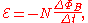which indicates that the induced emf (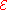) and the change in flux (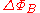) have opposite signs.

For a rigorous mathematical treatment, see electromagnetic induction
Electromagnetic induction
Electromagnetic induction is the production of an electric current across a conductor moving through a magnetic field. It underlies the operation of generators, transformers, induction motors, electric motors, synchronous motors, and solenoids....

and Maxwell's equations
Maxwell's equations
Maxwell's equations are a set of partial differential equations that, together with the Lorentz force law, form the foundation of classical electrodynamics, classical optics, and electric circuits. These fields in turn underlie modern electrical and communications technologies.Maxwell's equations...

.

## Opposing currents

If the magnetic field of current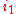induces another current
Current
- Science and Mathematics :* Electric current* Current , including** Ocean currents** Air currents** Current - currents in rivers and streams* Current density, mathematical concept unifying electric current, fluid current, and others...

,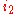, the direction ofis opposite that of. If these currents are in two circular conductors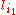and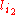respectively, then the currentsandmust counter-rotate. The opposing currents will repel each other as a result.

### Example

Currents bound inside the atoms of strong magnets can create counter-rotating currents in a copper or aluminum pipe. This is done by dropping the magnet through the pipe. When done, the descent of the magnet is observably slower than when dropped outside the pipe.

## Detailed interaction of charges in these currents

In electromagnetism, when charges change positions along electric field lines, work is done on them, whether it involves storing potential energy (negative work) or increasing kinetic energy (positive work).

When net positive work is applied to a charge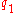, it gains momentum. The net work onthereby generates a magnetic field whose strength (in units of magnetic flux density (1 Tesla
Tesla (unit)
The tesla is the SI derived unit of magnetic field B . One tesla is equal to one weber per square meter, and it was defined in 1960 in honour of the inventor, physicist, and electrical engineer Nikola Tesla...

= 1 volt-second per square meter)) is proportional to the speed increase of. This magnetic field can interact with a neighboring charge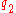, passing on this momentum to it, and in return,loses momentum.can also act onin a similar manner, by which it returns some of the emf that it received from. This back-and-forth component of emf contributes to magnetic inductance
Inductance
In electromagnetism and electronics, inductance is the ability of an inductor to store energy in a magnetic field. Inductors generate an opposing voltage proportional to the rate of change in current in a circuit...

. The closer thatandare, the greater the effect. Whenis inside a conductive medium such as a thick slab made of copper or aluminum, it more readily reacts to the emf sent to it by. The energy ofis not "instantly" consumed only as heat generated by the current ofbut is also stored in two opposing magnetic fields. The energy density of magnetic fields tends to vary by the square of the magnetic field's intensity; however, in the case of magnetically non-linear materials such as ferromagnets and superconductors, this relationship breaks down.

### Field energy

The electric field stores energy. The energy density of the electric field is given by: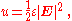In general the incremental amount of work per unit volume δW needed to cause a small change of magnetic field δB is: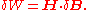### Conservation of momentum

Momentum must be conserved in the process, so ifis pushed in one direction, thenought to be pushed in the other direction by the same force at the same time. However, the situation becomes more complicated when the finite speed of electromagnetic wave propagation is introduced (see retarded potential
Retarded potential
The retarded potential formulae describe the scalar or vector potential for electromagnetic fields of a time-varying current or charge distribution. The retardation of the influence connecting cause and effect is thereby essential; e.g...

). This means that for a brief period of time, the total momentum of the two charges are not conserved, implying that the difference should be accounted for by momentum in the fields, as speculated by Richard P. Feynman. Famous 19th century electrodynamicist James Clerk Maxwell
James Clerk Maxwell
James Clerk Maxwell of Glenlair was a Scottish physicist and mathematician. His most prominent achievement was formulating classical electromagnetic theory. This united all previously unrelated observations, experiments and equations of electricity, magnetism and optics into a consistent theory...

called this the "electromagnetic momentum", although this idea is not generally accepted as a part of standard curricula in physics classes as of 2010. Yet, such a treatment of fields may be necessary in the case of applying Lenz's law to opposite charges. It is normally assumed that the charges in question are like charges. If they are not, such as a proton and an electron, the interaction is different. An electron generating a magnetic field would generate an emf that causes a proton to change its motion in the same direction as the electron. At first, this might seem to violate the law of conservation of momentum, but of course, such an interaction indeed conserves momentum once taking into account the momentum of electromagnetic fields.